## Interacting particle systems, (stochastic) PDEs, sandpiles, and fractals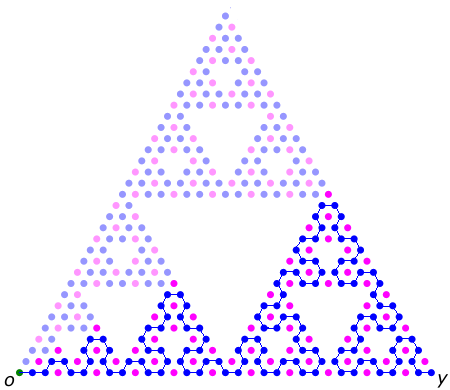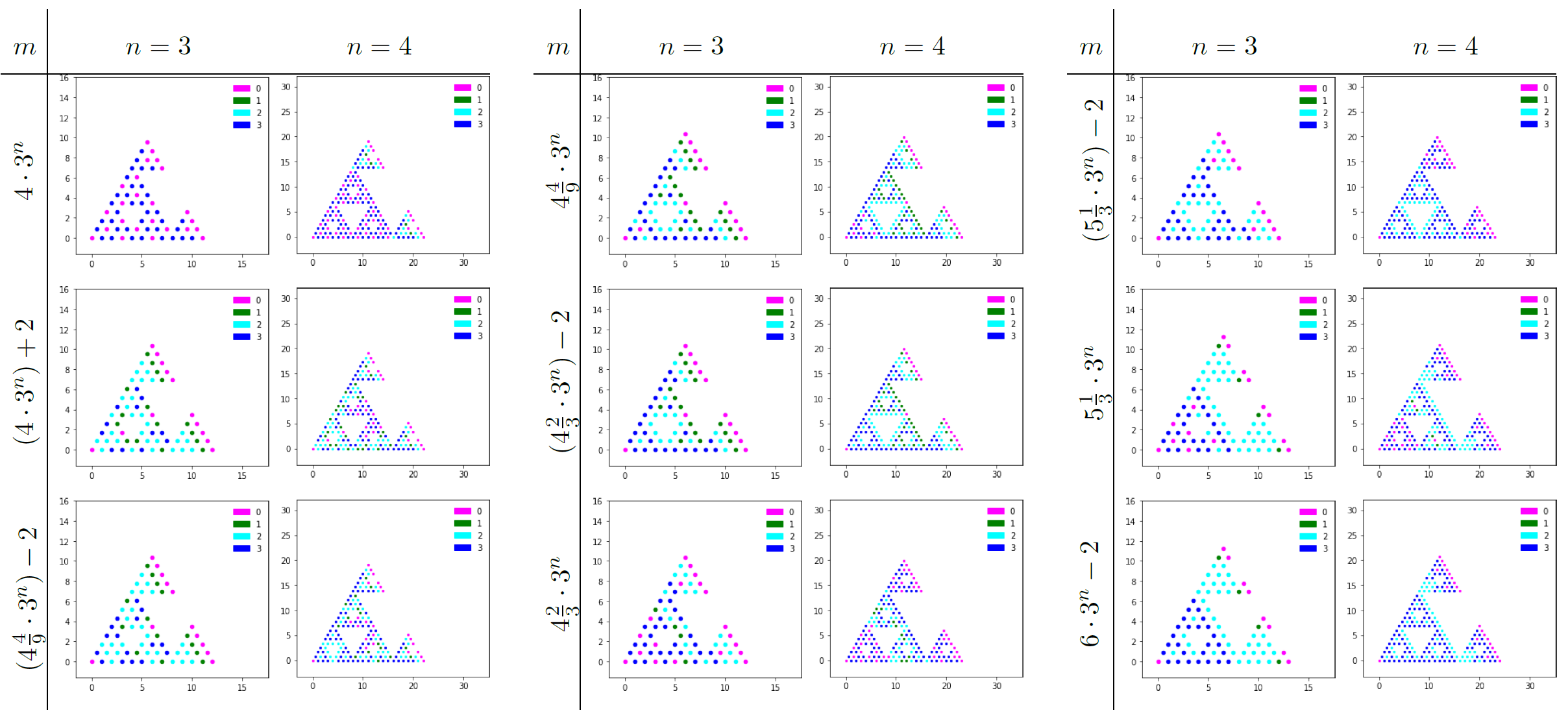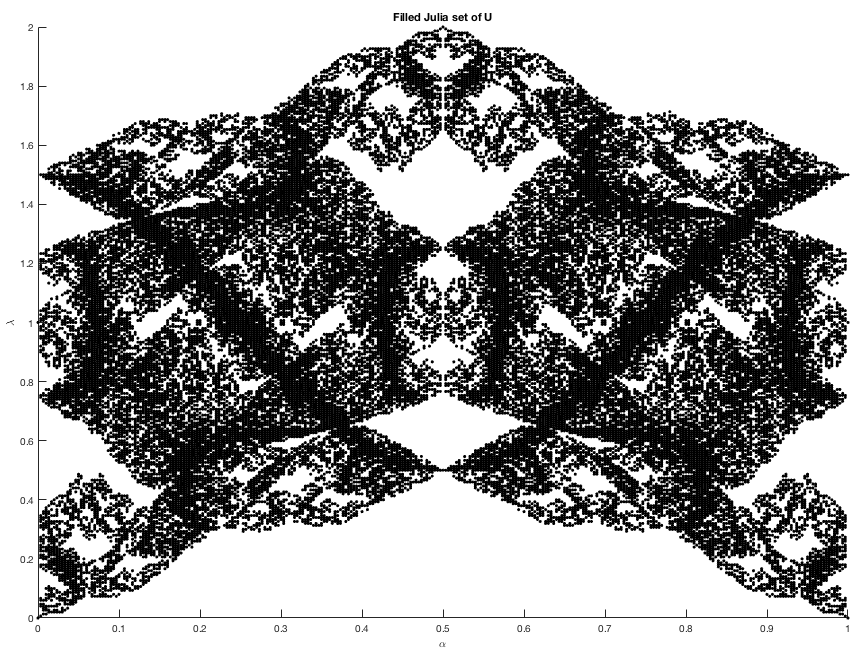##### Click on each image for a high-res version. From left to right: The identity element of the sandpile group of the level-5 Sierpinski gasket graph with 2 sinks. (Blue = 3, magenta = 0.) Observe the concatenation of the Sierpinski arrowhead curves in blue! The "periodic table" of abelian sandpile growth on the Sierpinski gasket, started from the bottom-left corner vertex. A "Hofstadter butterfly" on the Sierpinski gasket, which can be approximated by the filled Julia set of a 3-parameter dynamical system on $$(\mathbb{R}/\mathbb{Z})^2 \times \mathbb{R}$$.

My research specialty surrounds the analysis of probability models---in particular, interacting particle systems and Laplacian growth models---on state spaces which are bounded in distance determined by electrical resistance. By studying explicit models and taking the right space-time scaling limits, I can rigorously derive (stochastic) partial differential equations or prove limit shape theorems on these spaces. These mathematical results capture various laws of nature, such as heat flow, wave propagation, charged particles in an electromagnetic field, and fluid dynamics.

My current focus project is to establish microscopic derivations of (non)linear (S)PDEs from interacting particle systems---the main model being the (weakly a)symmetric exclusion process with variable speed boundary---on spaces which are bounded in the resistance metric. The two tunable "knobs" are the bulk antisymmetric jump rates and the rates of the boundary birth-and-death dynamics. By tuning these knobs we obtain a large variety of (S)PDEs with appropriate boundary conditions, describing the macroscopic behavior of particles in and out of equilibrium. The underlying state spaces include trees, fractals, and random environments, and do not necessarily possess translational invariance like Euclidean spaces. See papers 9, 11, 15, 16, P1, P2, P3.

Other probability models I have studied include: internal diffusion-limited aggregation (13), rotor-router aggregation (14), abelian sandpiles (14), and Gaussian free fields (3). In all of these models I have established optimal or near-optimal results on the corresponding state spaces. For instance, paper 14 is the first to rigorously establish that abelian sandpile growth on a geometrically self-similar state space exhibits power law modulated by log-periodic oscillations.

In order to gain a deeper understanding of stochastic processes on resistance spaces, I have also studied spectral analysis of Laplacian and Schrödinger operators on self-similar fractal spaces, on which there are a number of peculiar features (4, 5, 17). The results have implications for wave propagation (7), and for the asymptotic complexity of combinatorial structures such as spanning trees (10) and cycle-rooted spanning forests (17).

My paper 9 is an example where these two aspects of my work (probability theory + spectral analysis) meet: spectral gap inequality + electric network reduction = moving particle lemma in the exclusion process.

While the models I study may seem disparate, my approach is ultimately grounded in the study of analysis on graphs (Laplacian, heat kernels, and spectral analysis), Markov processes (such as random walks, Markov chains, Poisson processes, and Brownian motions), and potential theory (using techniques such as Dirichlet forms and related functional inequalities).

Papers 1, 6, 8, 14, 17 involved notable contributions from undergraduate students in the form of numerical findings, conjectures, and lemmas, which were then turned into theorems by the senior author(s).

As of July 1, 2019, my research is supported in part by the National Science Foundation (NSF) DMS Probability program grant DMS-1855604, "Stochastic dynamics on large-scale networks" (2019-22).

I also acknowledge recent support from the Simons Foundation (via a Collaboration Grant for Mathematicians, 2017-19) and the Research Council of Colgate University (Major grant 2017-18, Picker Research Fellowship 2019-20).

• Spectral decimation of the magnetic Laplacian on the Sierpinski gasket (AMS Sectional Meeting @ Binghamton U, Oct '19, written and delivered by Ruoyu Guo '19).
• Nonequilibrium fluctuations in the boundary-driven exclusion process on a resistance space (Stochastic Processes & their Applications conference @ Northwestern University, July '19).
• Random walks, electric networks, moving particle lemma, and hydrodynamic limits (Leiden Most Informal Probability Seminar, May '19).
• Laplacian growth and sandpiles on the Sierpinski gasket (UChicago Probability Seminar, Apr '19).
• [VIDEO] An abstract version of Bohr’s semiclassical asymptotic formula, with application to fractal spaces (Slightly dated, BIRS-CMO Oaxaca, Sep ‘15).

• I work actively with postdocs and PhD students* from around the world. As indicated above, my current research focus is on the analysis of interacting particle systems on high-dimensional, rough, or random environments. An ideal collaborator would be someone who is knowledgeable in probability theory and analysis of PDEs, and has mastered the basics behind the analysis of exclusion / zero-range process at the level of Kipnis & Landim, Scaling Limits of Interacting Particle Systems (Chapters 1-6 + Appendix A).

I no longer work on fractals per se, other than the fact that my work tackles problems concerning interacting particle systems on rough environments such as fractals. I would be happy to teach anyone about potential analysis & Dirichlet forms, but the collaborator would ideally complement me by having stronger experience with microscopic aspects of interacting particle systems (e.g. algebraic/stochastic duality), so that our discussions can weave back and forth between microscopic details and macroscopic limits.

Current postdoc and PhD student collaborators: Chiara Franceschini (Tecnico Lisboa), Rodrigo Marinho (Tecnico Lisboa), Otavio Menezes (Purdue), Federico Sau (IST Austria).

*Please note that Colgate University is a US liberal arts college, and does not offer a PhD program.

I work with select Colgate undergraduate students on challenging problems in probability and mathematical analysis that interface with physics (statistical physics, quantum physics) or theoretical computer science (randomized algorithms). While the topics look disparate, everything for me comes down to careful understanding of the Laplacian---generalization of the 2nd derivative in calculus, and more profoundly, the infinitesimal generator of a diffusion process---and the calculus thereof (e.g. integration by parts).

From experience, I look for students who not only have excelled in 300+-level MATH courses in the above-mentioned subjects, but also---more importantly---have the aptitude for independent problem-solving (analytical or numerical).

⚠ I am a mathematician, and my job is to come up with potential theorems and prove them logically.  Therefore I look for students whose mathematical ability is strong, whether or not they know how to program.  (Of course these two abilities are not mutually exclusive.) While I appreciate proficient programmers, the best outcomes occur with students who can contribute to theorem proving.

Please look below for research projects which resulted in arXiv preprints and then published in highly ranked journals.

## Research papers (see also my pages on arXiv, MathSciNet, zbMATH, ORCID)

### Papers in preparation

P1. Non-equilibrium and stationary density fluctuations in the boundary-driven exclusion process on the Sierpinski gasket, with Chiara Franceschini, Patrícia Gonçalves, and Otávio Menezes. Version 0.9, 36 pages, arXiv soon.
This paper is a continuation of 16, and the focus is on characterizing the non-equilibrium density fluctuations when the 3 reservoir rates are not identical. More precisely, under diffusive scaling we establish: the hydrostatic limit for the stationary measures of the discrete processes; and subsequential convergence of non-equilibrium density fluctuations, which can be upgraded to full convergence to an Ornstein-Uhlenbeck process if the initial-time fluctuations are centered Gaussian. From this we show that at stationarity, the fluctuation about the mean density profile $$\bar\rho(\cdot)$$ is a centered Gaussian field whose covariance has density $$\bar\rho(x)(1-\bar\rho(x)) 1_{\{x=y\}}- \frac{2}{3} \int_{SG} \, 2{\sf G}^{(2)}((z,z),(x,y)) \,d\Gamma(\bar\rho)(z)$$ with respect to the product self-similar measure on $$SG\times SG$$. Unlike the 1D case, it is unclear how to interpret the second term above, the convolution of the Green's function $${\sf G}^{(2)}$$ on the diagonal of $$SG\times SG$$ with the energy measure $$d\Gamma(\bar\rho)$$ on $$SG$$.
To prove all the above, we need to carefully estimate the two-point correlation functions in the exclusion process, and bring inputs from analysis on fractals, Dirichlet forms, and $$\Gamma$$-calculus.

P2. Cutoffs for exclusion processes on finite graphs with open boundaries, with Milton Jara and Rodrigo Marinho. 24+ pages, coming soon in Summer 2020
The $$\varepsilon$$-mixing time of an ergodic Markov chain is the first time the total variation distance between the chain distribution and the stationary distribution becomes less than $$\varepsilon$$. Given a sequence of Markov chains with $$\varepsilon$$-mixing times $$\{t_N^{\rm mix}(\varepsilon)\}_N$$, we say that they exhibit cutoff if there exists a sequence of time scales $$\{t_N\}_N$$ such that for every $$\varepsilon\in (0,1)$$, $$t_N^{\rm mix}(\varepsilon) / t_N \to 1$$. In this paper we prove a general theorem on cutoffs for the symmetric exclusion processes on graphs with open boundaries, under the natural assumption that the graphs converge geometrically and spectrally to a compact metric measure space with Dirichlet boundary condition. Our theorem is valid on a variety of settings including, but not limited to, the discrete hypercube in $$\mathbb{R}^d$$ for every dimension $$d$$; self-similar fractal spaces; and products thereof. The proof is by construction of a "cutoff martingale," the analysis thereof using $$L^2$$-spectral methods, and a coupling between dual exclusion processes.E1. Potential theory of diagonal-reflected random walks. Expository note, Version 0.96, 13+ pages.
P3. Macroscopic density fluctuations in the exclusion process with variable-speed boundary: From 1D to the Sierpinski gasket.
This paper gives a non-technical summary and interpretation of the main results from 16 and P1, and also extends the analysis of P1 to mixed boundary reservoir dynamics.

P4. Cutoffs for exclusion and interchange processes on finite graphs, with Rodrigo Marinho. 10+ pages, coming soon in Summer 2020.
In this paper we prove a general theorem on cutoffs for the symmetric exclusion process and the interchange process on graphs with no boundary (torus) or with closed (Neumann) boundaries. The theorem works in any dimension.

P5. Scaling limits of fluctuations in weakly asymmetric exclusion processes on resistance spaces.

### Submitted

17. Spectral decimation of the magnetic Laplacian on the Sierpinski gasket: Solving the Hofstadter-Sierpinski butterfly, with Ruoyu (Tony) Guo. 46 pages, revised version submitted (2020).
In this paper we provide a recursive construction of the magnetic spectrum on the Sierpinski gasket under uniform magnetic field, thereby solving the "Hofstadter-Sierpinski spectral problem" in its entirety. The renormalization map is a 3-parameter map involving non-rational functions. (As far as we know, this is the first time spectral decimation has been carried out with non-rational functions. We succeeded in carrying out the decimation in all scenarios, including the exceptional set for spectral decimation.) In special cases, we can also compute determinants of the magnetic Laplacians on the gasket, which induce a random spatial process called the cycle-rooted spanning forest (CRSF) process, cf. Rick Kenyon's 2011 Annals of Probability paper.

16. Asymptotic behavior of density in the boundary-driven exclusion process on the Sierpinski gasket, with Patrícia Gonçalves. 44 pages, submitted (2019+).
In this paper we prove a LLN and a CLT for the particle density in the boundary-driven exclusion process on the Sierpinski gasket. Depending on the strength of the boundary reservoirs' dynamics, the particle density evolves according to the heat equation with Dirichlet, Robin, or Neumann boundary condition. In the equilibrium setting, we prove that the density fluctuations satisfy the Ornstein-Uhlenbeck equation with the corresponding boundary condition.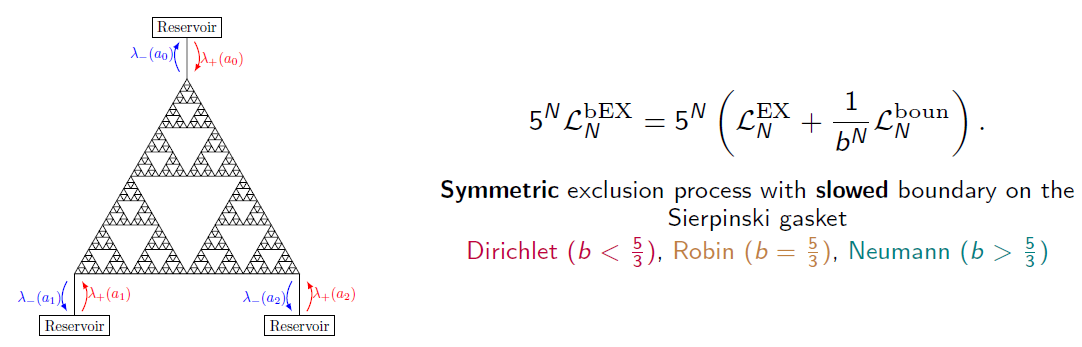15. Local ergodicity in the exclusion process on an infinite weighted graph. 36 pages, submitted (2018+).
In this paper I prove, on every strongly recurrent weighted graph, the coarse-graining arguments needed to pass from the microscopic observables (in the exclusion process) to the corresponding macroscopic averages. The two-blocks estimate is based on the moving particle lemma established in paper 9.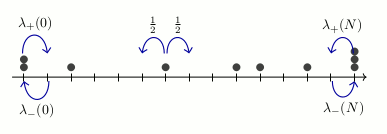### 2020+

14. Laplacian growth and sandpiles on the Sierpinski gasket: limit shape universality & exact solutions, with Jonah Kudler-Flam. 54 pages, to appear in Ann. Inst. Henri Poincaré Comb. Phys. Interact. (2020+). Click here for Jonah's AutomataSG program and animations on GitHub.
In this paper we solve the rotor-router aggregation problem and the abelian sandpile growth problem on the graphical Sierpinski gasket (SG) when particles are launched from the corner vertex. The sandpile growth problem is solved exactly via a renormalization scheme involving self-similar sandpile tiles. In the process we also prove the identity elements of the sandpile groups of subgraphs of SG (with two different boundary conditions).

Conclusion: The four Laplacian growth models---IDLA, rotor-router aggregation, divisible sandpiles, and abelian sandpiles---started from the corner vertex of SG satisfy "limit shape universality" in the sense of Levine and Peres (2017).### 2020

13. Internal DLA on Sierpinski gasket graphs, with Wilfried Huss, Ecaterina Sava-Huss, and Alexander Teplyaev. Chapter 7 in "Analysis and Geometry on Graphs & Manifolds," M. Keller et al. (Eds.), London Mathematical Society Lecture Notes, Cambridge University Press (2020).
In this paper we prove that starting from a corner vertex of SG, an internal diffusion-limited aggregation process (where successive i.i.d. random walks deposit upon first exit from the previous cluster) fills balls in the graph metric with probability 1.See also the shape theorem for divisible sandpiles on SG by Huss and Sava-Huss.

12. Fractal AC circuits and propagating waves on fractals, with Eric Akkermans, Gerald Dunne, Luke G. Rogers, and Alexander Teplyaev. 9 pages, Chapter 18 in "Analysis, Probability, and Mathematical Physics on Fractals," P. Alonso-Ruiz et al. (Eds.), World Scientific Publishing (2020).

### 2018

11. From non-symmetric particle systems to non-linear PDEs on fractals, with Michael Hinz and Alexander Teplyaev. In A. Eberle et al. (Eds.), Stochastic Partial Differential Equations and Related Fields: In Honor of Michael Röckner SPDERF, Bielefeld, Germany, October 10 -14, 2016, Springer Proceedings in Mathematics & Statistics (2018), pp. 503-513.

10. Regularized Laplacian determinants of self-similar fractals, with Alexander Teplyaev and Konstantinos Tsougkas. Lett. Math. Phys. 108 (2018) 1563-1579. (See also correction: pp. 1581-1582.)In this paper we give rigorous meaning to regularized logarithmic Laplacian determinants on several fractal graphs, using properties of the spectral zeta function. In two examples (the double-sided SG and the double-sided pq model) we find that the logarithmic discrete graph Laplacian determinant has a leading-order term whose coefficient is the asymptotic complexity constant in the enumeration of spanning trees, and whose lagging term corresponds to the regularized logarithmic Laplacian determinant. Our results generalize those of Chinta, Jorgenson, and Karlsson on the discrete tori, and have implications for quantum fields and statistical mechanics on fractals.

### 2017

9. The moving particle lemma for the exclusion process on a weighted graph. Electron. Commun. Probab. 22 (2017), paper no. 47.I prove in this paper a Sobolev-type energy inequality for the exclusion process, which is analogous to Thomson's (or Dirichlet's) principle for random walks / electric networks. It builds upon the marvelous "octopus inequality" proved by Caputo, Liggett, and Richthammer in 2009.

8. Power dissipation in fractal AC circuits, with Luke G. Rogers, Loren Anderson, Ulysses Andrews, Antoni Brzoska, Aubrey Coffey, Hannah Davis, Lee Fisher, Madeline Hansalik, Stephen Loew, and Alexander Teplyaev. (2015 UConn math REU fractals group) J. Phys. A: Math. Theor. 50 325205 (2017)We give a "fractal spin" on the classic infinite ladder circuit discussed in the Feynman Lectures on Physics. For the Feynman-Sierpinski ladder circuit (pictured) we can rigorously prove the convergence of the effective impedances using the dynamics of Möbius transformations.
A refinement of our results (concerning the energy measure) on the F-S ladder circuit was attained recently by Patricia Alonso-Ruiz.

7. Wave equations on one-dimensional fractals with spectral decimation and the complex dynamics of polynomials, with Ulysses Andrews, Grigory Bonik, Richard W. Martin, and Alexander Teplyaev. J. Fourier Anal. Appl. 23 (2017) 994-1027. Click here for the wave animations described in the paper.
We study wave propagation on the pq-model, a self-similar inhomogeneous wave medium on the unit interval, with an initial approximate delta pulse at the origin. Using the spectral resolution of the Laplacian (via spectral decimation), we prove that the solution of the wave equation can be approximated uniformly in space by a Fourier series, up to some cutoff time. This gives a quantitative glimpse into the "infinite speed of wave propagation" phenomenon on fractals.

6. Stabilization by Noise of a $$\mathbb{C}^2$$-Valued Coupled System, with Lance Ford, Derek Kielty, Rajeshwari Majumdar, Heather McCain, Dylan O'Connell, and Fan Ny Shum. (2015 UConn math REU stochastics group) Stoch. Dyn. 17 (2017) 1750046.
In this paper we study a coupled ODE system in two complex dimensions that admits finite-time blow-up solutions. We show analytically and numerically that stabilization can be achieved by adding a suitable Brownian noise, and that the resulting system of SDEs is ergodic. The proof uses Girsanov theorem to effect a time change from our 2D system to a quasi-1D-system similar to the one studied by Herzog and Mattingly.

### 2016 and prior

5. Singularly continuous spectrum of a self-similar Laplacian on the half-line, with Alexander Teplyaev. J. Math. Phys. 57 052104 (2016).
4. Spectral dimension and Bohr's formula for Schrodinger operators on unbounded fractal spaces, with Stanislav Molchanov and Alexander Teplyaev. J. Phys. A: Math. Theor. 48 395203 (2015). (JPhysA Cover image)
3. Entropic repulsion of Gaussian free field on high-dimensional Sierpinski carpet graphs, with Baris Evren Ugurcan. Stoch. Proc. Appl. 125 (2015) 4632-4673.
2. Periodic billiard orbits of self-similar Sierpinski carpets, with Robert Niemeyer. J. Math. Anal. Appl. 416 (2014) 969-994.
1. Quantum Theory of Cavity-Assisted Sideband Cooling of Mechanical Motion, with Florian Marquardt, Aashish Clerk, and Steven M. Girvin. Phys. Rev. Lett. 99 093902 (2007).

### Books edited

B1. Analysis, Probability and Mathematical Physics on Fractals. Patricia Alonso-Ruiz, Joe P. Chen, Luke G. Rogers, Robert S. Strichartz, and Alexander Teplyaev (Eds.). World Scientific Publishing (2020).

Back to JPC's homepage